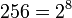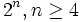# Groups of order 256

## Contents

See pages on algebraic structures of order 256| See pages on groups of a particular order

## Statistics at a glance

Note that since prime power order implies nilpotent, and$256 = 2^8$ is a prime power, all groups of order 256 are nilpotent.

Quantity Value Greatest integer function of logarithm of value to base 2 Explanation for value
Number of groups up to isomorphism 56092 15
Number of abelian groups up to isomorphism 22 4 Equal to the number of unordered integer partitions of 8, see classification of finite abelian groups
Number of groups of class exactly two up to isomorphism 31742 14
Number of groups of class exactly three up to isomorphism 21325 14
Number of groups of class exactly four up to isomorphism 2642 11
Number of groups of class exactly five up to isomorphism 320 8
Number of groups of class exactly six up to isomorphism 38 5
Number of groups of class exactly seven up to isomorphism, i.e., maximal class groups 3 1 classification of finite 2-groups of maximal class. For order$2^n, n \ge 4$, there are exactly three maximal class groups: dihedral, semidihedral, and generalized quaternion. For order 256, the groups are: dihedral group:D256, semidihedral group:SD256, and generalized quaternion group:Q256.